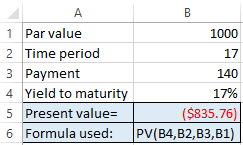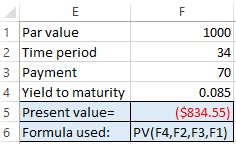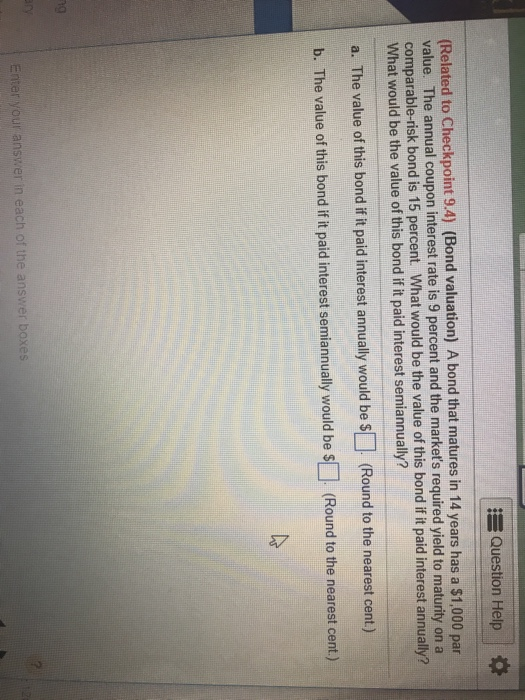Question

# A bond that matures in 17 years has a ​\$1 000 par value. The annual coupon...

A bond that matures in 17 years has a ​\$1 000 par value. The annual coupon interest rate is 14 percent and the​ market's required yield to maturity on a​ comparable-risk bond is 17 percent.

What would be the value of this bond if it paid interest​ annually? ______

What would be the value of this bond if it paid interest​ semiannually? _______

Calculation for annual interest payment:

Par value is \$1000
Time period is 17 years
Annual coupon rate is 14%
Annual coupon payment =(Coupon rate)*(Par value)=(14%)*(1000)=140
Yield to maturity=17%

We can determine the present value using excel as:Answer: If the interest is paid annually, then the value of the bond will be equal to \$835.76.

Calculation for semiannual interest payment:

Par value is \$1000
Time period is 17 years, as the interest is paid semiannually, the number of periods=17*2=34

Semi annual coupon payment=(Annual coupon payment)/2=140/2=70
Annual yield to maturity=17%
Semiannual yield to maturity=17%/2=0.085
We can determine the present value using excel as:Answer: If the interest is paid semi annually, then the value of the bond will be equal to \$834.55

#### Earn Coins

Coins can be redeemed for fabulous gifts.

Similar Homework Help Questions
• ### A bond that matures in 17 years has a ​\$1000 par value. The annual coupon interest...

A bond that matures in 17 years has a ​\$1000 par value. The annual coupon interest rate is 15 percent and the​ market's required yield to maturity on a​ comparable-risk bond is 14 percent. What would be the value of this bond if it paid interest​ annually? What would be the value of this bond if it paid interest​ semiannually? Question 7-3

• ### A bond that matures in 13 years has a ​\$1,000 par value. The annual coupon interest...

A bond that matures in 13 years has a ​\$1,000 par value. The annual coupon interest rate is 8 percent and the​ market's required yield to maturity on a​ comparable-risk bond is 16 percent. What would be the value of this bond if it paid interest​ annually? What would be the value of this bond if it paid interest​ semiannually?

• ### ​(Bond valuation) A bond that matures in 19 years has a ​\$1,000 par value. The annual...

​(Bond valuation) A bond that matures in 19 years has a ​\$1,000 par value. The annual coupon interest rate is 14 percent and the​ market's required yield to maturity on a​ comparable-risk bond is 13 percent. What would be the value of this bond if it paid interest​ annually? What would be the value of this bond if it paid interest​ semiannually? a.  The value of this bond if it paid interest annually would be ​\$ nothing. ​(Round to the...

• ### A bond that matures in 13 years has a \$1,000 par value. The annual coupon interest...

A bond that matures in 13 years has a \$1,000 par value. The annual coupon interest rate is 7 percent and the markets required yield to maturity on a comparable risk bond is 15 percent. What would be the value of this bond if it paid interest annually? What would be the value of this bond if it paid interest semiannually?

• ### A bond that matures in 12 years has a \$1,000 par value. The annual coupon interest rate is 11 percent and the​ market's...

A bond that matures in 12 years has a \$1,000 par value. The annual coupon interest rate is 11 percent and the​ market's required yield to maturity on a​ comparable-risk bond is 17 percent. What would be the value of this bond if it paid interest​ annually_____? What would be the value of this bond if it paid interest​ semiannually_____? a.  The value of this bond if it paid interest annually would be ​\$nothingm. ​(Round to the nearest​ cent.)

• ### A bond that matures in 12 years has a \$1,000 per value. The annual interest rate...

A bond that matures in 12 years has a \$1,000 per value. The annual interest rate is 12 percent and the market's yield to maturity on a comparable-risk bond is 14 percent. What would be the value of this bond if it paid interest annually? What would be the value of this bond if it paid if it paid interest semiannually?w

• ### Calculate the value of a bond that matures in 12 years and has a \$1,000 par...

Calculate the value of a bond that matures in 12 years and has a \$1,000 par value. The annual coupon interest rate is 14 percent and the​ market's required yield to maturity on a​ comparable-risk bond is 12 percent. The value of the bond is_____?

• ### Calculate the value of a bond that matures in 14 years and has a \$1,000 par...

Calculate the value of a bond that matures in 14 years and has a \$1,000 par value. The annual coupon interest rate is 13 percent and the​ market's required yield to maturity on a​ comparable-risk bond is 15 percent. Question 7-2

• ### (1) (Bond Valuation) a bond that matures in 9 years has a \$1000 par value. the...

(1) (Bond Valuation) a bond that matures in 9 years has a \$1000 par value. the annual coupon interest rate is 14% and the markets required yield to maturity on a comparable risk-bond is 16%. what would be the value of this bond if it paid interest annually? what would be the vale of this bond if it paid interest semi-annually? (2) (yield to maturity) the market price is \$850 for a 12-year bond (\$1000 par value) that pays 9%...

• ### EQuestion Help (Related to Checkpoint 9.4) (Bond valuation) A bond that matures in 14 years has...EQuestion Help (Related to Checkpoint 9.4) (Bond valuation) A bond that matures in 14 years has a \$1,000 par value. The annual coupon interest rate is 9 percent and the market's required yield to maturity on a comparable-risk bond is 15 percent. What would be the value of this bond if it paid interest annually? What would be the value of this bond if it paid interest semiannually? a. The value of this bond if it paid interest annually would...